# NCERT Solutions 9th Maths, Science, Social Science, Hindi 2020

NCERT Solutions 9th Class Syllabus and Subjects 2019 to 2020, Class 9 Maths Class 9 Science Class 9 Social Science Class 9 Hindi Download The Subject Wise NCERT Solutions For 9th Standard

 S. No.
 Ncert solutions for class 9 science Subjects wise Details 2019-2020
 1
 MATTER IN OUR SURROUNDINGS
 2
 IS MATTER AROUND US PURE
 3
 ATOMS AND MOLECULES
 4
 STRUCTURE OF THE ATOM
 5
 THE FUNDAMENTAL UNIT OF LIFE
 6
 TISSUES
 7
 DIVERSITY IN LIVING ORGANISMS
 8
 MOTION
 9
 FORCE AND LAWS OF MOTION
 10
 GRAVITATION
 11
 WORK  AND ENERGY
 12
 SOUND
 13
 WHY DO WE FALL ILL
 14
 NATURAL RESOURCES
 15
 IMPROVEMENT IN FOOD RESOURCES

EXERCISE 13.8
Assume π =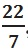, unless stated otherwise.
1. Find the volume of a sphere whose radius is
(i) 7 cm                            (ii) 0.63 m
Solution: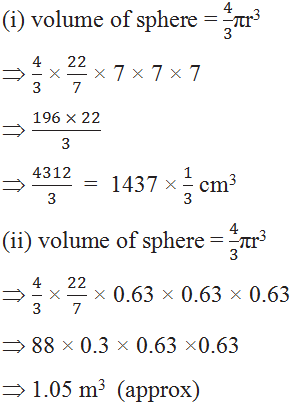2. Find the amount of water displaced by a solid spherical ball of diameter
(i) 28 cm                                     (ii) 0.21 m
Solution: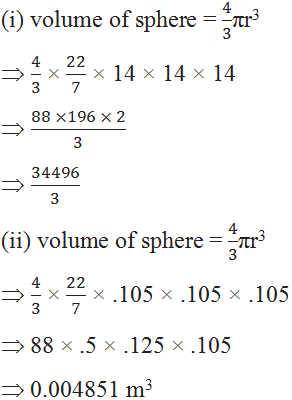3. The diameter of a metallic ball is 4.2 cm. What is the mass of the ball, if the density of the metal is 8.9 g per cm3 ?
Solution: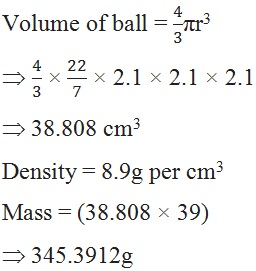4. The diameter of the moon is approximately one-fourth of the diameter of the earth. What fraction of the volume of the earth is the volume of the moon?
Solution:
Let diameter of earth = x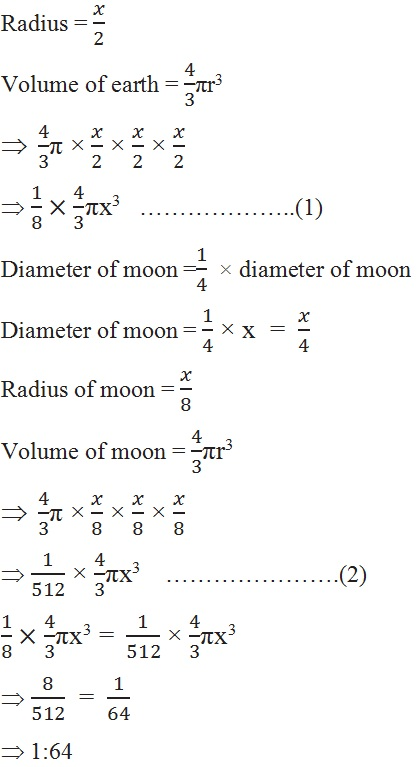5. How many litres of milk can a hemispherical bowl of diameter 10.5 cm hold?
Solution:
Diameter = 10.5 ,  radius = 5.25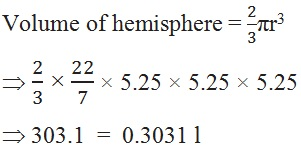6. A hemispherical tank is made up of an iron sheet 1 cm thick. If the inner radius is 1 m, then find the volume of the iron used to make the tank.
Solution: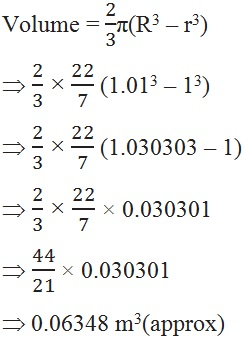7. Find the volume of a sphere whose surface area is 154 cm2.
Solution:
Surface area = 154 cm2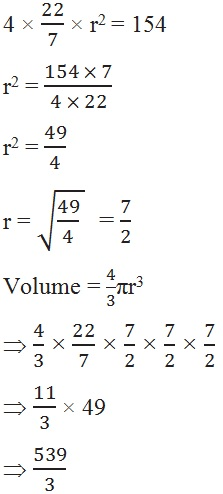8. A dome of a building is in the form of a hemisphere. From inside, it was white-washed at the cost of 498.96. If the cost of white-washing is 2.00 per square metre, find the
(i) inside surface area of the dome, (ii) volume of the air inside the dome.
Solution: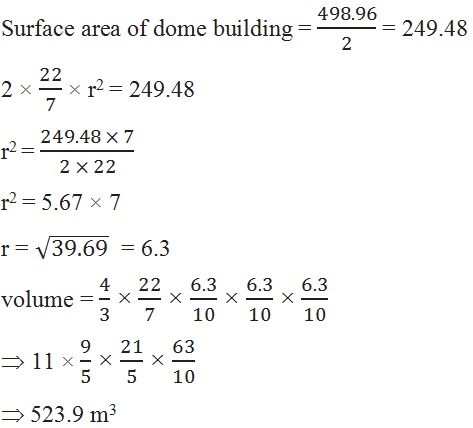9. Twenty seven solid iron spheres, each of radius r and surface area S are melted to form a sphere with surface area S2. Find the
(i) radius r2 of the new sphere,                  (ii) ratio of S and S2.
Solution:
R = r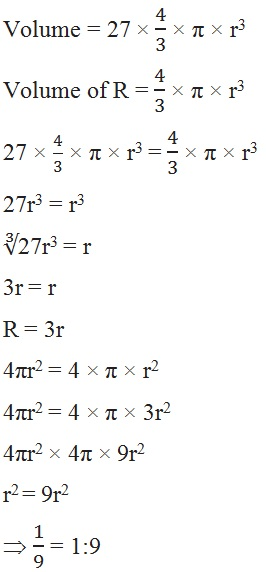10. A capsule of medicine is in the shape of a sphere of diameter 3.5 mm. How much medicine (in mm3) is needed to fill this capsule?
Solution :
Diameter = 3.5 mm ,  r = 1.75 mm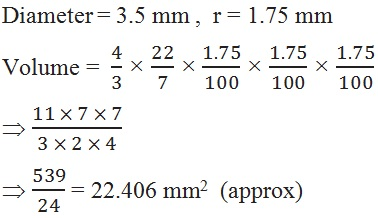*****